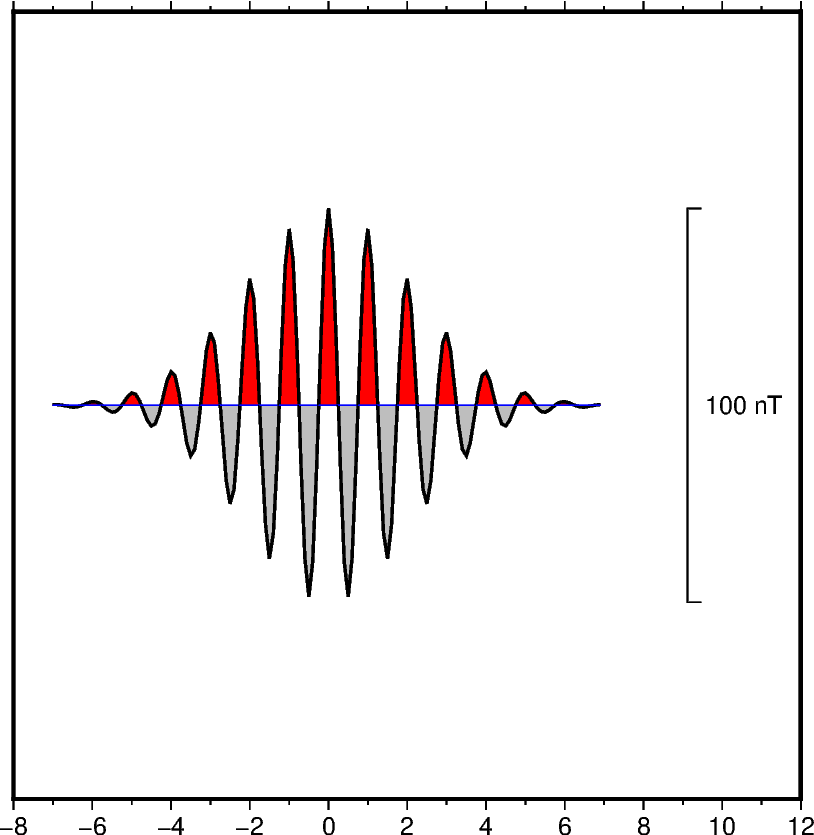# Wiggle along tracks

The `pygmt.Figure.wiggle` method can plot z = f(x,y) anomalies along tracks. `x`, `y`, `z` can be specified as 1d arrays or within a specified file. The `scale` parameter can be used to set the scale of the anomaly in data/distance units. The positive and/or negative areas can be filled with color by setting the `color` parameter.Out:

```<IPython.core.display.Image object>
```

```import numpy as np
import pygmt

# Create (x, y, z) triplets
x = np.arange(-7, 7, 0.1)
y = np.zeros(x.size)
z = 50 * np.exp(-((x / 3) ** 2)) * np.cos(2 * np.pi * x)

fig = pygmt.Figure()
fig.basemap(region=[-8, 12, -1, 1], projection="X10c", frame=["Snlr", "xa2f1"])
fig.wiggle(
x=x,
y=y,
z=z,
# Set anomaly scale to "20c"
scale="20c",
# Fill positive and negative areas red and gray, respectively
color=["red+p", "gray+n"],
# Set the outline width to "1.0p"
pen="1.0p",
# Draw a blue track with a width of 0.5 points
track="0.5p,blue",
# Plot a vertical scale bar at the right middle. The bar length is 100 in
# data (z) units. Set the z unit label to "nT".
position="jRM+w100+lnT",
)
fig.show()
```

Total running time of the script: ( 0 minutes 0.938 seconds)

Gallery generated by Sphinx-Gallery